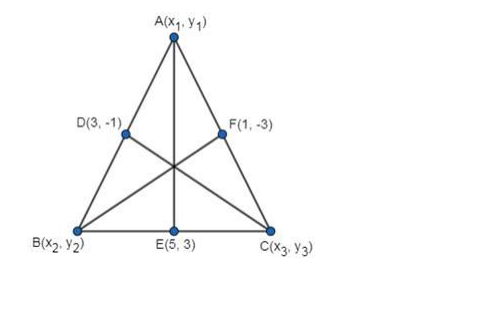Deepak Scored 45->99%ile with Bounce Back Crack Course. You can do it too!

# Find the area of ΔABC, the midpoints of whose sides AB,

Question:

Find the area of ΔABC, the midpoints of whose sides AB, BC and CA are D(3, -1), E(5, 3) and F(1, -3) respectively.

Solution:The figure is as shown above.

$x_{1}+x_{2}=2 \times 3=6 \ldots .(1)$

$x_{1}+x_{3}=2 \times 1=2 \ldots \ldots(2)$

$x_{2}+x_{3}=2 \times 5=10 \ldots \ldots(3)$

Equation 1 - Equation 2 gives us

$\mathrm{X}_{2}-\mathrm{X}_{3}=4$ ..........(4)

Equation 3 + Equation 4,

$2 x_{2}=14 \Rightarrow x_{2}=7$

$\therefore \mathrm{x}_{1}=-1$ and $\mathrm{x}_{3}=3$

Similarly,

$y_{1}+y_{2}=2 \times-1=-2 \ldots \ldots(5)$

$y_{1}+y_{3}=2 \times-3=-6 \ldots . .(6)$

$y_{2}+y_{3}=2 \times 3=6 \ldots . .(7)$

Equation 5 – Equation 6 gives us

$\mathrm{y}_{2}-\mathrm{y}_{3}=4 \ldots .(8)$

Equation 7 + Equation 8,

$2 y_{2}=10 \Rightarrow y_{2}=5$

$\therefore \mathrm{y}_{1}=-7$ and $\mathrm{y}_{3}=1$

Area of $\Delta A B C=\frac{1}{2}\left[x_{1}\left(y_{2}-y_{3}\right)+x_{2}\left(y_{3}-y_{1}\right)+x_{3}\left(y_{1}-y_{2}\right)\right]$

$=\frac{1}{2}[-1(5-1)+7(1+7)+3(-7-5)]$

$=\frac{1}{2}[-4+56-36]$